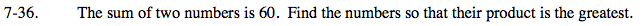### Home > PC > Chapter 7 > Lesson 7.2.1 > Problem7-36

7-36.Let x = 1st number
Let y = 2nd number
Let P = the product
x + y = 60
P = xy

1. Solve the first equation for y and substitute the result in the second equation.
2. Check where the graph of P is a maximum.